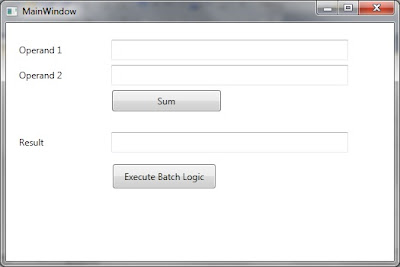## Thursday, February 24, 2011

### Rx - Executing anonymous code asynchronously

In the previous post we discuss about how we can execute a method asynchronously using Reactive Extensions. In this post we will discuss how we can execute anonymous code asynchronously using Reactive Extensions. Rx provides this support using Observable.Start.

Invoking Actions (No data returned):
Let us start with discussing how we can execute code asynchronously with no data to return.The event handler of Click event of the button is as follows:
`private void button2_Click(object sender, RoutedEventArgs e){    Observable.Start(() =>        {            Thread.Sleep(2000);            return;        }    )    .ObserveOnDispatcher()    .Subscribe(                (result) => { ;},                (ex) => this.Background = Brushes.Red,                () => this.Background = Brushes.Green);}`

This code just executes simple code asynchronously in a ThreadPool thread. It generates OnNext, OnCompleted and OnError messages on the Dispatcher thread as we are observing on Dispatcher. After atleast two seconds the background of window should turn green.

Invoking anonymous code (data returned from lambda) asynchronously:
We can also execute anonymous asynchronous code using Observable.Start which actually return a value. This code is in the form of lambda expressions or lambda statements which explicitly return data through return statement. Let us add a button on the same window. When user clicks the button it should update the operand boxes with any random number.
`private void btnFillRandomOperands_Click(object sender, RoutedEventArgs e){    Observable.Start<int>(        () =>        {            Thread.Sleep(2000);            return new Random().Next(1, 10);        }).ObserveOnDispatcher<int>()        .Subscribe(                       (result) =>                       {                           this.textBoxOperand1.Text = result.ToString();                           this.textBoxOperand2.Text = result.ToString();                       },                        (ex) => this.Background = Brushes.Red,                        () => this.Background = Brushes.Green                    );}`

The above code uses Random class to generate a random number between 1 and 10. The returned number is provided to OnNext handler by Rx runtime. This handler updates both operand text boxes with this number. After this, the runtime places OnCompleted handler which turns the background of the window to green.

When we run this and click this button, it results in the following window after atleast 2 seconds. Definitely, it might be a different random number generated and filled in the operands text boxes.Passing Arguments to Code executed by Observable.Start:
It doesn’t seem that we can have any parameters to Observable.Start directly. But we can use our old technique of using Captured Variables. As we know that lambdas can capture variables from the scope they are generated from. The variables used by lambda like this are called Captured variables. Like a regular lambda statement executed asynchronously, these variables also have Closure issues. I have discussed here how to avoid closure issues by assigning the value of captured variables to local variables inside lambdas.

http://shujaatsiddiqi.blogspot.com/2010/12/wpf-dispatcherbegininvoke-and-closures.html
`private void button1_Click(object sender, RoutedEventArgs e){    decimal operand1 = Decimal.Parse(this.textBoxOperand1.Text);    decimal operand2 = Decimal.Parse(this.textBoxOperand2.Text);    Observable.Start(() =>                         {                             Thread.Sleep(2000);                             return operand1 + operand2;                         })                         .ObserveOnDispatcher()                         .Subscribe(                                (result) => { this.textBoxResult.Text = result.ToString(); });    operand1 = 3;    operand2 = 4;}`

In the above case what do you guess would be shown in the result textbox if we enter 2.1 and 3.5 in the two operand text boxes. If you guess 5.6 then you would be surprised to see this result.This is the result of modified captured variables as the modified value of those captured variables are used which are 3 and 4. The result of addition of 3 and 4 is 7, which is displayed in the Result text box. In order to fix this, we can define two new local variables localOperand1 and localOperand2 and initialize them with the values of captured variables.
`private void button1_Click(object sender, RoutedEventArgs e){    decimal operand1 = Decimal.Parse(this.textBoxOperand1.Text);    decimal operand2 = Decimal.Parse(this.textBoxOperand2.Text);    Observable.Start(() =>                         {                             decimal localOperand1 = operand1;                             decimal localOperand2 = operand2;                             Thread.Sleep(2000);                              return localOperand1 + localOperand2;                         })                         .ObserveOnDispatcher()                         .Subscribe(                                (result) => { this.textBoxResult.Text = result.ToString(); });    Thread.Sleep(2);    operand1 = 3;    operand2 = 4;}`

When we run the application and enter data 2.1 and 3.5 then the correct result is displayed in the Result text box.Although Resharper still shows warning but it is just fine as we have seen the correct result being calculated.Observable.Start and Exceptions in the code executed:
As a result of an exception Observable.Start behaves same way as Observable.ToAsync. We can represent the behavior in marble diagram as follows:Let us update the code executed asynchronously using Observable.Start so that if the sum of number entered is either zero or lesser, it should throw an Exception.
`[DebuggerStepThrough]private void button1_Click(object sender, RoutedEventArgs e){    decimal operand1 = Decimal.Parse(this.textBoxOperand1.Text);    decimal operand2 = Decimal.Parse(this.textBoxOperand2.Text);    Observable.Start(() =>    {        decimal localOperand1 = operand1;        decimal localOperand2 = operand2;        decimal sum = localOperand1 + localOperand2;        Thread.Sleep(2000);        if (sum <= 0)        {            throw new System.Exception("Error in computation");        }        return sum;    })    .ObserveOnDispatcher()    .Subscribe(        (result) => { this.textBoxResult.Text = result.ToString(); },        (ex) => this.Background = Brushes.Red,        () => this.Background = Brushes.Green);}`

You can notice that we are still subscribing on Dispatcher. In addition to OnNext, we have provided the code for OnCompleted and OrError. The window should turn green when the operation completed successfully resulting in OnCompleted. In case of exception, OnError is executed.Using IScheduler to run Asynchronous code:
Like Observable.ToAsync, Observable.Start also provides overloads to run the asynchronous code using different IScheduler. We can run code which returns or does not return any data in the form of lambda and we can run it using the IScheduler of our choice. You can see that just by providing Scheduler.Dispatcher info to Start we can turn this method to run on UI thread:
`Observable.Start(() =>    {        Thread.Sleep(2000);        return;    }, Scheduler.Dispatcher)`

Note:
There is one more overload of Observable.Start which returns ListObservable<TSource>. Since it is not about executing anonymous asynchronous code, we are discussing it here.